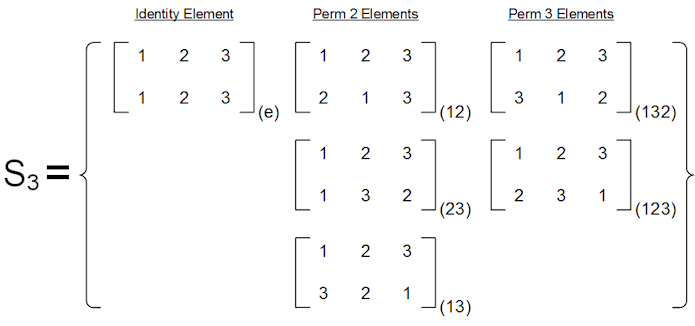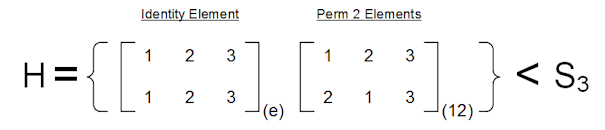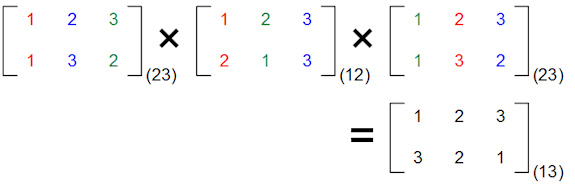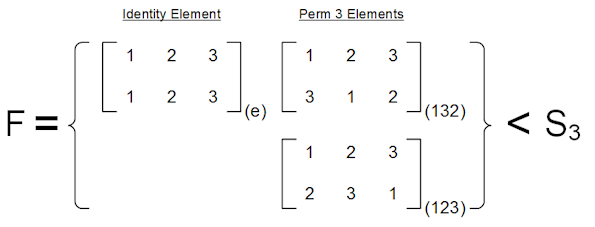# 9 – Normality

 < ρℝεν | ℂσητεητs | ℕεχτ >We have normality. I repeat, we have normality. Anything you still can’t cope with is therefore your own problem. – Douglas Adams, The Hitchhiker’s Guide to the Galaxy

In the last Chapter, we introduced the concept of Subgroups, or Groups within a Group, and considered some examples. In what may have appeared as an aside, we examined the decomposition of Natural Numbers into multiples of Prime Numbers. We also rather informally talked about Simple Groups, our loose definition being those Groups which have no Subgroups. As ever, things are not so… well, simple. In this Chapter, we present the formal definition of a Simple Group; something that requires the introduction of the concept of a Normal Subgroup. Leveraging this new concept, and extending the analogy of Prime Decomposition, we will also begin to consider how to decompose Groups into more fundamental constituents, a process which will culminate in a fundamental property of Groups covered in Chapter 10.

What Passes for Normal Round HereSo this is going to be a little technical, rather than shying away from this, let’s jump right in. For a Group B with a Subgroup A, A is said to be a Normal Subgroup of B, written:

A ◁ B

if it fulfils an additional property, namely:

For every element a ∈ A and b ∈ B

bab-1 ∈ A

That is if you take any element of the Normal Subgroup, pre-multiply it by any element of the Group and post-multiply it by the inverse of this same element, the result is another member of the Normal Subgroup.

One immediate result of this definition is that all Subgroups of an Abelian Group (one whose binary operation is commutative) are Normal Subgroups.

This is because xy = yx for all members of the Group. Which in turn means that:

bab-1 = bb-1a = ea = a

The definition of a Normal Subgroup may seem rather artificial, but the concept is an important one and we will come across one of the reasons why in a little while. I can now also properly restate my definition of a Simple Group. A Simple Group is a Group with no Normal Subgroups.

Before looking more at this concept, I’ll explore some examples of Subgroups and determine whether or not they are normal.

Let us first consider GL2(ℝ), the Group of 2 × 2 invertible matrices with entries in the Real Numbers, under the operation of matrix multiplication. We met a Subgroup of this earlier, the one consisting of the set of diagonal 2 × 2 matrices. Let’s denote the Subgroup by D2(ℝ), though this is not standard nomenclature . Does this pass the normality test? If we consider a generic element of GL2(ℝ), a matrix A with entries {a, b}{c, d} then back in Chapter 5 we defined its inverse A-1 to be:$\begin{bmatrix}a&b\\c&d\end{bmatrix}^{-1}=\dfrac{1}{ad-bc}\begin{bmatrix}d&-b\\-c&a\end{bmatrix}$

If we consider a generic diagonal matrix, D with entries {x, 0}{0, y} then we need to evaluate ADA-1. Using our definition of a generic inverse, we can do this as follows:$\begin{bmatrix}a&b\\c&d\end{bmatrix}\times\begin{bmatrix}x&0\\0&y\end{bmatrix}\times\dfrac{1}{ad-bc}\begin{bmatrix}d&-b\\-c&a\end{bmatrix}=$$\begin{bmatrix}ax&by\\cx&dy\end{bmatrix}\times\dfrac{1}{ad-bc}\begin{bmatrix}d&-b\\-c&a\end{bmatrix}=$$\dfrac{1}{ad-bc}\begin{bmatrix}(axd-byc)&(-axb+bya)\\(cxd-dyc)&(-cxb+dya)\end{bmatrix}$

In general, the resulting matrix is not a member of D2(ℝ), but we can also see that of we set x = y, then the minor diagonal elements cancel and we get:$\dfrac{1}{ad-bc}\begin{bmatrix}(axd-bxc)&0\\0&(-cxb+dxa)\end{bmatrix}=\begin{bmatrix}x&0\\0&x\end{bmatrix}$

Which is the same matrix we started with. So, if we call this restricted group D*2(ℝ), we can say:

D*2(ℝ) ◁ GL2(ℝ)

For a counter example, let’s think about the Permutation Group of degree 3, S3. This is the Group arising from rearrangement of a set of size three. If we denote the three elements of such as set by {1, 2, 3} then we can use the same sort of approach we adopted in Chapter 3 to define members of S3 by mapping out what happens to each of {1, 2, 3} (the earlier example used coloured circles, using numbers is equivalent, they are just labels). This results in the following Group:It is worth pointing out what the numbers in round brackets next to each permutation mean. They are an abbreviation of the permutation. So (12) may be read as 1 goes to 2 and 2 goes to 1 (the last element loops back to the first one) and (123) can be read as 1 goes to 2, 2 goes to 3 and 3 goes to 1. This is a more economic way of writing the same permutations. In a formulation like (23) where 1 is not mentioned, it is assumed that 1 is unchanged by the permutation.

Let’s now consider a Subgroup of S3 which consists of just the identity element and the permutation (12) and call this H. The longhand version of H is shown below:First of all we can see that this a Subgroup because (12)(12) = e implying both closure and that (12)-1 = (12). Is it a Normal Subgroup?

Let’s use the element of S3 denoted by (23) as our example. As above (23)-1 = (23), so we want to determine:

Is (23)(12)(23) ∈ H?

Working this through longhand:In the above exhibit the colour coding helps to see what happens to the original numbers. So if we pick the red text. The first permutation sends 1 to 1 (no change). The second permutation takes 1 to 2. The final one takes the 2 from the second permutation to 3. So overall we get 1 going to 3. The blue and green colours track similar paths of permutations strung together.

It is worth noting here that we can use the shorthand notation in a similar way to tracing through the coloured items in the longer version. So if we consider (23)(12)(23), we can deduce:

Nothing happens to one in the first permutation (23)

1 goes to 2 in the second first permutation (12)

2 goes to 3 in the final permutation (23)

so overall 1 goes to 3, which means our final permutation starts (13…)

Next:

2 goes to 3 in the first permutation (23)

Nothing happens to 3 in the second permutation (12)

3 goes to 2 in the final permutation (23)

so overall 2 is unchanged, which means that 2 will not appear in our final permutation

Finally:

3 goes to 2 in the first permutation (23)

2 goes to 1 in the second permutation (12)

Nothing happens to 1 final permutation (23)

so overall 3 goes to 1, which means we can complete the resulting permutation, the answer is (13)

Having shown (by either of the routes used above) that the resulting permutation is (13), we can also see that:

(23)(12)(23) = (13) ∉ H 

So H is not a Normal Subgroup of S3.

Let’s instead consider the Subgroup consisting of the identity element and the two permutations of three elements. Denote this set by F, its members are as follows:This is clearly a Subgroup . As all the permutations of three elements are included in F, we don’t need to check pre- and post-multiplying by these to establish normality. So focusing on the permutations of two elements (which are their own inverses) we see:

(12)(123)(12) = (132)

(12)(132)(12) = (123)

similar logic holds for (23) and (13)

So this time we have:

F ◁ S3 

Hopefully the above examples help to illustrate the concept of a Normal Subgroup. Let’s now put these entities to a different use.

The Deciding FactorI must again apologise in advance for a somewhat technical section coming up. In my defence we have already met some Quotient Groups, albeit that I didn’t call out this fact at the time. These were specifically Groups like ℤn formed by looking at addition Modulo n on the Integers, ℤ .

n is equivalently the Quotient Group  created by considering the type of Subgroups of ℤ which we mentioned earlier in this Chapter, namely {nx where n ∈ ℤ}, so all even numbers (2ℤ), all multiples of 3 (3ℤ) and so on. In a sense ℤ4 is ℤ “divided by” the set of all integer multiples of 4, or ℤ/4ℤ.

This is the essence of a Quotient Group, which is created by using a Normal Subgroup to split a Group into parts. The resulting parts, taken together, can be used to define a new Group. While not 100% the same, the process is analogous to dividing 12 by 3 to get 4 as we might when trying to factor 12 into constituent prime numbers. If you get that and feel you don’t need to know more about this area, then please skip past the two boxes below and pick up our story again later.

 Cosets of Subgroups If you are still with me, then let’s step back and first of all introduce the concept of a coset, which is rather more simple that then name might suggest. For a Subgroup H of a Group G and an element g ∈ G we define the coset of H in G with respect to g as: gH = {gh such that h ∈ H} This means that we create a coset of H in G with respect to g by multiplying each element of H by g in turn and gathering together the results in a set, which we label gH. Actually that is the left coset. There is also a right coset similarly defined by: Hg = {hg such that h ∈ H} Of course in an Abelian Group, these two are identical as gh = hg. However they are also identical in non-Abelian Groups so long as ghg-1 ∈ H for all g ∈ G and for all h ∈ H. This is precisely our definition of a Subgroup being Normal (I told you it would come in useful later). Turning this round the other way, if H ◁ G then Hg = gH for all g ∈ G. That is, for a Normal Group, left and right cosets are the same. A Subgroup being Normal and a Subgroup having its left and right cosets being identical are two ways of saying precisely the same thing. There are entire branches of Mathematics in which left an right cosets are not equivalent. I’m generally going to ignore them and instead mostly focus on the situation where they are the same. If nothing else, this allows me to drop “left” and “right” and just say “coset”. We are able to do this iff (recall that terminology from earlier?) H is a normal Subgroup, so let’s assume that it is. What does a coset actually look like? Let’s return to our example of S3 with the set elements labelled {1, 2, 3} as before. Using the shorthand we introduced earlier, S3 consists of: e, the identity element (i.e. no permutations) 3 permutations which rearrange only two of the set members: (12), (13), (23) and 2 permutations which rearrange all three of the set members: (123), (132) Earlier we showed the that Subgroup F = {e, (123), (132)} ◁ S3, let’s look at some cosets arising from this Normal Subgroup using different members of S3. (12)F = {(12)e, (12)(123), (12)(132)} Working through the compound permutations we can set that: (12)F = {(12), (13), (23)} Using similar logic: (13)F = {(12), (13), (23)} and (23)F = {(12), (13), (23)} So each of (12)F, (13)F and (23)F yield the same coset. Then also: (123)F = {(123)e, (123)(123), (123)(132)} Which means that: (123)F = {(123), (132), e} And similarly: (132)F = {(132), (123), e} These cosets are also identical, so we actually have only two cosets of F in S3: {(12), (13), (23)} {e, (123), (132)} We can see that the latter is F itself and that the former is (12)F [or equivalently either (13)F or (23)F]. As a second example of this process, let’s up the stakes and consider S4 the Group arising from the permutations of a set of four elements. Remembering our rule that Sn has n! elements, where: n! = 1 × 2 × 3 × … × n-1 × n We can see that S should have 24 members (1 × 2 × 3 × 4 = 24). Let’s list them. I’ll continue to to use the notation where (132) means 1 goes to 3, 3 goes to 2 and 2 goes to 1), this approach yields members of S4 as follows:Most of these are relatively easy to figure out: Obviously we have the identity element (no rearrangements) There are 6 permutations of two elements There are 8 permutations of three elements There are 6 permutations of four elements What might escape attention initially (this being something which does not occur in smaller sizes of Sn) is the final three elements: There are 3 pairs of permutations of two elements Which gets us to our total Group size of 24. In Chapter 8 I mentioned that S3 was clearly a Subgroup of S4 because S3 would be equivalent to all the permutations in S4 which leave one of the four set members unchanged. I have illustrated this in the diagram above by picking out the six permutations which do not change 4. These are highlighted in white. It will be evident that these are the same S3 with which we have become familiar . We can use this Subgroup – let’s just call it S3 – to form left cosets  as we did in the last example. Some obvious candidates for the elements of S4 which we might try first are the permutations of two elements which include 4. I.e.: (14) × S3 (24) × S3 (34) × S3 This would give us the following cosets to work out:If we work out all of the combined permutations, we get the following 4 cosets:We should be able to stop at this point because we know that 4 × 6 = 24 and so we must have covered all elements of S4. Multiplying by any other element of S4 should just reproduce the same cosets (as we saw above with cosets of F = {e, (123), (132)} in S3. However, there is something amiss here compared to our previous result with S3. If we idly look at S3 × (14) instead of (14) × S3 we get the following:It is obvious that this is not the same as any of the other cosets we generated above. What has gone wrong? What happened to the nice tidiness of the S3 example? The reason for this is that S3 is not a Normal Subgroup of S4, just a regular Subgroup. We can see this by considering the action of a member of S4 which is not in S3 on a member of S3. If we note that the inverse of a permutation of two items, say (14), is the same permuation, i.e. (14)-1 = (14), then: (14)(123)(14)-1 = (423) ∉ S3 So, by definition, S3 is not a Normal Subgroup of S4. In general, if our Subgroup is not Normal, we get this type of unruly behaviour. All the Subgroups I’ll use going forward will be Normal, but it is worth remembering the above and to always proceed with appropriate caution when working with Subgroups. So we have seen two examples of decomposing a Group into cosets. The first used a Normal Subgroup and was well behaved, the second didn’t and we ran into issues. Let’s build on these results and try to be more generic in the next box.
 Using Cosets to create Quotient Groups If we assume that H is a Normal Subgroup of G. We have defined what is meant by a coset of H in G for a specific g ∈ G. What happens when g ranges through all of G. In particular, can we talk about the set of all cosets of H in G as we have above for F in S3? Yes we can and, as in the first example we worked through above (but not – let’s remember – as in the second example), this is defined as: G/H = {gH where g ∈ G} So if we form all possible cosets (left or right, it doesn’t matter as H is Normal) and collect these together as a set labelled G/H, this new entity is a Quotient Group. However, as we have emphasised many times, a Group is a combination of a set and a binary operator. How are we to combine two cosets? The definition is as follows: (gH)(fH) = (gf)H For H being normal (and only for H being normal) this is well-defined because: (gH)(fH) = g(Hf)H If we use the equivalence of Hf and fH then: g(Hf)H = g(fH)H = (gf)HH = (gf)H  Using this definition, is G/H a Group? Closure Using our definition of the binary operator: if gH and fH are cosets, then: (gH)(fH) = (gf)H As G is a Group and g, f ∈ G, gf ∈ G (G must be closed) So (gf)H is also a coset   Identity H itself seems like a good candidate here, particularly if we note that we can write it as eH (where e is the identity element of G). We then have: (eH)(gH) = (eg)H = gH   Inverses For any g ∈ G there must also exist g-1 ∈ G. If we form the coset of H in G with respect to g-1, we get: (gH)(g-1H) = (gg-1)H = eH = H   Associative Because we have defined the binary operator on G/H in terms of multiplying elements of G and because the latter operation is associative, so is G/H. So G/H is indeed a Group. Let’s go back to our worked example of F = {e, (123), (132)} ◁ S3. We have: D3/F = {F, (12)F} Using our defined binary operator for Quotient Groups, we can see that: F * F = F F * (12)F = (12)F (12)F * (12)F = F This structure should be pretty familiar, it is precisely ℤ2 or equivalently C2.

In the second of the two boxes above we demonstrated that, for a given Group and one of its Normal Subgroups, we can generate a Quotient Group. In our worked example we showed that we can decompose S3 (which we recall is of order 6) into a Normal Subgroup isomorphic to ℤ3 and a Quotient Group (created by “dividing” S3 by our Normal Subgroup) isomorphic to ℤ2. So we have:

S3 Is of size 6

F ◁ S3 Is of size 3

F is isomorphic to ℤ3

S3/F is of size 2

S3/F Is isomorphic to ℤ2

We have decomposed S3/F in to Normal Subgroup F isomorphic to ℤ3 and a resulting Quotient Group S3/F isomorphic to ℤ2

We mentioned above that if n is prime then ℤn is simple. Both 2 and 3 are prime and so both ℤ2 and ℤ3 simple Groups. So we have broken S3 down into Simple Subgroups, a concept we will be returning to very shortly.

To close this Chapter, a couple of numeric points. First it may be becoming obvious that the size of a Subgroup (and thus a Normal Subgroup) must be a divisor of the size of the Group. We write this as:

If H < G then |H| is a divisor of |G| 

Where – as before |X| denotes “the size of set X”.

Second, it is also worth noting that it is no coincidence that 6 = 3 × 2 is the decomposition of 6 in to prime factors.

In the final Chapter of this set of three, I’ll used the results we have obtained so far to go back to the key question of the decomposition of Groups into Subgroups.

 Concepts Introduced in this Chapter Normal Subgroup A Subgroups A of a Group G whose members also satisfy the property that gag-1 ∈ A for all a ∈ A and g ∈ G. Simple Group Formal definition of the concept introduced in Chapter 7a. A Simple Group is one with no Normal Subgroups. Abelian Subgroups All Subgroups of an Abelian Group are Normal. Cosets Structures created by multiplying the elements of a Subgroup A of a Group G by other elements of G. If the Subgroup is Normal, then it doesn’t matter if we pre- or post-multiply, we get the same Cosets. If the Subgroup is not Normal, then the left and right cosets will be different. Quotient Group A Group defined by Normal Subgroup A of a Group G and consisting of all cosets of A in G. This is written as G/A. Forming a Quotient Group is somewhat analogous to dividing a Natural Number by some divisor. We split G into a number of subsets, each of the size of the Normal Group, but modified by some multiplying element. Group Decomposition Informally this is the process by which a Group is split into sub-elements of smaller degree.
 < ρℝεν | ℂσητεητs | ℕεχτ >

Chapter 9 – Notes

  It is rather too close to Dn which as we know is the Dihedral Group of degree n.  It is probably obvious – by applying the Ghostbusters Theorem – that ∉ is the opposite of ∈.  Given the isomorphism between S3 and D3, the set of reflections and rotations of an equilateral triangle, that we established in Chapter 3, we can see that F is isomorphic to the set of rotations of an equilateral triangle, a Subgroup of D3. This Subgroup is isomorphic to the Cyclic Group of order 3, C3. As an aside, when a Mathematician says “clearly” they generally mean that they can’t be bothered to do the work involved in making it clear!  Following for on from the last footnote, our isomorphism also means that the rotations of an equilateral triangle form a Normal Subgroup of D3.  Indeed I’ve also played rather fast and loose with my terminology a more accurate label for these Groups would be ℤ/nℤ.  Quotient Groups are also often referred to as Factor Groups.  It should be noted that this Subgroup does not arise from the choice of 4 (though this makes the Subgroup more obvious). If we had decided to pick out all permutations which keep 1 constant, we would still get S3, just with a different set of permutations: S3 = {e, (23), (24), (34), (234), (243)} An even easier way to see this is to realise that {1, 2, 3, 4} are just labels – we used colours to define S3 the first time we met it in Chapter 3. So using {2, 3, 4} leads to the same Permutation Group as {1, 2, 3}, as does {A, B, C} or – as previously mentioned – {Goose, Fox, Bag of Grain/Corn}.  The reason that I have reintroduced the word “left” will become apparent soon.  The last step, (gf)HH = (gf)H, works because we have already shown that H is closed.  This result (Lagrange’s Theorem) also shows than any Group whose size is p, a prime number, must be a Simple Group; indeed, it must be isomorphic to the Cyclic Group of order p, Cp, which is also equivalent to ℤp.
 Text: © Peter James Thomas 2016-18. Images: © Peter James Thomas 2016-17, unless stated otherwise. Published under a Creative Commons Attribution 4.0 International License.